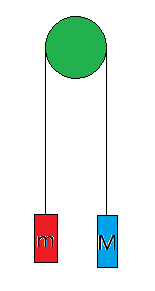# $\Large{\blue{With\space variable \space masses}}$In the above pulley mass system mass $m$ decreases with a constant rate of $w$.

At $time=0s,m=M\Rightarrow m=M-wt$

If $x,y$ are the horizontal distances of $m,M$ from the pulley respectively and $T$ is the tension in the string $\Rightarrow y+x=constant$ $\Rightarrow\dot{y}=-\dot{x}$ $\Rightarrow \ddot{y}=-\ddot{x}$ $Applying \space newton's \space second \space law \space on \space each \space mass$ $M\ddot{y}=Mg-T..........$ $\dfrac{dm\dot{x}}{dt}=mg-T$ $\Rightarrow \dfrac{dm\dot{y}}{dt}=T-mg..........$ $+\Rightarrow\dfrac{dm\dot{y}}{dt}+M\ddot{y}=(M-m)g$ $\Rightarrow \dfrac{d[(M-wt)\dot{y}]}{dt}+M\ddot{y}=wtg$ $\Rightarrow \int_{t=0}^{t=t_1}{d[(M-wt)\dot{y}]}+\int_{t=0}^{t=t_1}M\ddot{y}{dt}=\int_{t=0}^{t=t_1}wtg{dt}$ $\Rightarrow M\dot{y}+(M-wt_1)\dot{y}=\dfrac{wt_1^2g}{2}$ $Since \space we \space can \space replace \space t_1 \space with\space t$ $\Rightarrow\dot{y}=\dfrac{wt^2g}{2(2M-wt)}$ $\Rightarrow \triangle y=\dfrac{wg}{2}\int_{0}^{t_1}\dfrac{t^2}{2M-wt}dt$ $let \space I=\int\dfrac{t^2}{\red{a-bt}}\red{dt}$ $=-\int\dfrac{t^2}{b}\red{d\ln|a-bt|}\space\space\space\blue{\because\dfrac{dt}{a-bt}=-\dfrac{-d\ln|a-bt|}{b}}$ $=-\dfrac{1}{b}\left({t^2\ln|a-2b|} -2\int t\ln|a-bt|dt\right)$ $= \dfrac{1}{b}\left(\red{2\int t\ln|a-bt|dt}-{t^2\ln|a-2b|} \right)$ $=\dfrac{1}{b}\left(\red{J}-{t^2\ln|a-2b|} \right)$ $\dfrac{J}{2}=\int t\ln|a-bt|dt$ $\blue{\because d[x\ln|a-bt|]-\dfrac{a}{b}d\ln|a-bt|-dt=\ln|a-bt|dt}$ $\therefore \dfrac{J}{2}=\int td[x\ln|a-bt|] -\dfrac{a}{b}\int td\ln|a-bt|-\int tdt$ $=\left(t^2\ln|a-bt|-\red{\int t\ln|a-bt|dt}\right)-\dfrac{a}{b}\left(t\ln|a-bt|-\int \ln|a-bt|dt\right)-\dfrac{t^2}{2}+\blue{C}$ $\Rightarrow\red{\dfrac{J}{2}}=t^2\ln|a-bt|-\red{\dfrac{J}{2}}-\dfrac{a}{b}\left(\cancel{t\ln|a-bt|}-\left(\cancel{t}-\dfrac{a}{b}\right)\ln|a-bt|+t\right)-\dfrac{t^2}{2}+\blue{C}$ $\Rightarrow J=t^2\ln|a-bt|-\dfrac{a^2}{b^2}\ln|a-bt|-\dfrac{at}{b}-\dfrac{t^2}{2}+\blue{C}$ $\Rightarrow J=\left(t^2-\dfrac{a^2}{b^2}\right)\ln|a-bt|-\left(\dfrac{at}{b}+\dfrac{t^2}{2}\right)+\blue{C}$ $\Rightarrow I=\dfrac{1}{b}\left(\red{\left(\cancel{t^2}-\dfrac{a^2}{b^2}\right)\ln|a-bt|-\left(\dfrac{at}{b}+\dfrac{t^2}{2}\right)+{C}}-\cancel{t^2\ln|a-2b|} \right)$ $=\dfrac{-1}{b}\left(\dfrac{ a^2}{b^2}\ln| a-bt|+\dfrac{ at}{b}+\dfrac{t^2}{2}+{C} \right)$ $=\dfrac{-1}{w}\left(\dfrac{4M^2}{w^2}\ln|2M-wt|+\dfrac{2Mt}{w}+\dfrac{t^2}{2}+{C} \right)$ $\Rightarrow \triangle y=\dfrac{wg}{2}\red{[I]_{0}^{t}}$ $=\dfrac{\cancel{w}g}{2\cancel{w}}\left(\dfrac{4M^2}{w^2}\ln\left(\left|\dfrac{2M}{2M-wt}\right|\right) -\dfrac{2Mt}{w}-\dfrac{t^2}{2}\right)$ $\Rightarrow \boxed{\triangle y={g}\left(\dfrac{2M^2}{w^2}\ln\left(\left|\dfrac{2M}{2M-wt}\right|\right) -\dfrac{Mt}{w}-\dfrac{t^2}{4}\right)}$Note by Zakir Husain
3 months, 1 week ago

This discussion board is a place to discuss our Daily Challenges and the math and science related to those challenges. Explanations are more than just a solution — they should explain the steps and thinking strategies that you used to obtain the solution. Comments should further the discussion of math and science.

When posting on Brilliant:

• Use the emojis to react to an explanation, whether you're congratulating a job well done , or just really confused .
• Ask specific questions about the challenge or the steps in somebody's explanation. Well-posed questions can add a lot to the discussion, but posting "I don't understand!" doesn't help anyone.
• Try to contribute something new to the discussion, whether it is an extension, generalization or other idea related to the challenge.

MarkdownAppears as
*italics* or _italics_ italics
**bold** or __bold__ bold
- bulleted- list
• bulleted
• list
1. numbered2. list
1. numbered
2. list
Note: you must add a full line of space before and after lists for them to show up correctly
paragraph 1paragraph 2

paragraph 1

paragraph 2

[example link](https://brilliant.org)example link
> This is a quote
This is a quote
    # I indented these lines
# 4 spaces, and now they show
# up as a code block.

print "hello world"
# I indented these lines
# 4 spaces, and now they show
# up as a code block.

print "hello world"
MathAppears as
Remember to wrap math in $$ ... $$ or $ ... $ to ensure proper formatting.
2 \times 3 $2 \times 3$
2^{34} $2^{34}$
a_{i-1} $a_{i-1}$
\frac{2}{3} $\frac{2}{3}$
\sqrt{2} $\sqrt{2}$
\sum_{i=1}^3 $\sum_{i=1}^3$
\sin \theta $\sin \theta$
\boxed{123} $\boxed{123}$

Sort by:

$\Large{\boxed{Bonus\space Problem}}$ $\boxed{\red{What \space if\space w \space is\space not\space constant\space but\space instead \space is \space a\space function\space of\space time ?}}$ (Answer is in the reply)

- 3 months, 1 week ago

$\triangle y = g\int_{t=0}^{t=t_1}\dfrac{fdt}{2M-f'}$ $where\space f=\iint wdt dt$

- 3 months, 1 week ago

This is good mathematical physics. I miss doing proper physics and maths 😭 school takes a lot of my time

- 3 months ago

$\boxed{\Large{\purple{Bonus \space Problem}}}$ $\boxed{What\space if\space the \space pulley \space have \space a \space considerable \space moment \space of \space inertia\space = \space I ?}$

- 2 months, 3 weeks ago